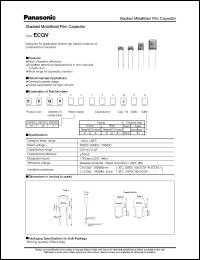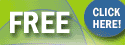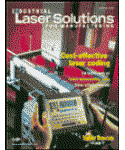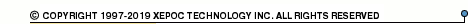More than4 307 195 components listedECQV series datasheets. Manufacturer: Panasonic (Matsushita).

 ECQV Designed for application where high density insertion of components is required. Datasheet*) ECQV1103JM Designed for application where high density insertion of components is required. Datasheet*) ECQV1104JM Designed for application where high density insertion of components is required. Datasheet*) ECQV1123JM Designed for application where high density insertion of components is required. Datasheet*) ECQV1124JM Designed for application where high density insertion of components is required. Datasheet*) ECQV1153JM Designed for application where high density insertion of components is required. Datasheet*) ECQV1154JM Designed for application where high density insertion of components is required. Datasheet*) ECQV1183JM Designed for application where high density insertion of components is required. Datasheet*) ECQV1184JM Designed for application where high density insertion of components is required. Datasheet*) ECQV1223JM Designed for application where high density insertion of components is required. Datasheet*) ECQV1224JM Designed for application where high density insertion of components is required. Datasheet*) ECQV1273JM Designed for application where high density insertion of components is required. Datasheet*) ECQV1274JM Designed for application where high density insertion of components is required. Datasheet*) ECQV1333JM Designed for application where high density insertion of components is required. Datasheet*) ECQV1334JM Designed for application where high density insertion of components is required. Datasheet*) ECQV1393JM Designed for application where high density insertion of components is required. Datasheet*) ECQV1394JM Designed for application where high density insertion of components is required. Datasheet*) ECQV1473JM Designed for application where high density insertion of components is required. Datasheet*) ECQV1474JM Designed for application where high density insertion of components is required. Datasheet*) ECQV1563JM Designed for application where high density insertion of components is required. Datasheet*) ECQV1683JM Designed for application where high density insertion of components is required. Datasheet*) ECQV1823JM Designed for application where high density insertion of components is required. Datasheet*) ECQV1H103JL Designed for application where high density insertion of components is required. Datasheet*) ECQV1H104JL Designed for application where high density insertion of components is required. Datasheet*) ECQV1H105JL Designed for application where high density insertion of components is required. Datasheet*) ECQV1H123JL Designed for application where high density insertion of components is required. Datasheet*) ECQV1H124JL Designed for application where high density insertion of components is required. Datasheet*) ECQV1H125JL Designed for application where high density insertion of components is required. Datasheet*) ECQV1H153JL Designed for application where high density insertion of components is required. Datasheet*) ECQV1H154JL Designed for application where high density insertion of components is required. Datasheet*) ECQV1H155JL Designed for application where high density insertion of components is required. Datasheet*) ECQV1H183JL Designed for application where high density insertion of components is required. Datasheet*) ECQV1H184JL Designed for application where high density insertion of components is required. Datasheet*) ECQV1H185JL Designed for application where high density insertion of components is required. Datasheet*) ECQV1H223JL Designed for application where high density insertion of components is required. Datasheet*) ECQV1H224JL Designed for application where high density insertion of components is required. Datasheet*) ECQV1H273JL Designed for application where high density insertion of components is required. Datasheet*) ECQV1H274JL Designed for application where high density insertion of components is required. Datasheet*) ECQV1H333JL Designed for application where high density insertion of components is required. Datasheet*) ECQV1H334JL Designed for application where high density insertion of components is required. Datasheet*) ECQV1H393JL Designed for application where high density insertion of components is required. Datasheet*) ECQV1H394JL Designed for application where high density insertion of components is required. Datasheet*) ECQV1H473JL Designed for application where high density insertion of components is required. Datasheet*) ECQV1H474JL Designed for application where high density insertion of components is required. Datasheet*) ECQV1H563JL Designed for application where high density insertion of components is required. Datasheet*) ECQV1H564JL Designed for application where high density insertion of components is required. Datasheet*) ECQV1H683JL Designed for application where high density insertion of components is required. Datasheet*) ECQV1H684JL Designed for application where high density insertion of components is required. Datasheet*) ECQV1H823JL Designed for application where high density insertion of components is required. Datasheet*) ECQV1H824JL Designed for application where high density insertion of components is required. Datasheet*) ECQV1J103JM Designed for application where high density insertion of components is required. Datasheet*) ECQV1J104JM Designed for application where high density insertion of components is required. Datasheet*) ECQV1J105JM Designed for application where high density insertion of components is required. Datasheet*) ECQV1J123JM Designed for application where high density insertion of components is required. Datasheet*) ECQV1J124JM Designed for application where high density insertion of components is required. Datasheet*) ECQV1J153JM Designed for application where high density insertion of components is required. Datasheet*) ECQV1J183JM Designed for application where high density insertion of components is required. Datasheet*) ECQV1J184JM Designed for application where high density insertion of components is required. Datasheet*) ECQV1J223JM Designed for application where high density insertion of components is required. Datasheet*) ECQV1J224JM Designed for application where high density insertion of components is required. Datasheet*) ECQV1J273JM Designed for application where high density insertion of components is required. Datasheet*) ECQV1J274JM Designed for application where high density insertion of components is required. Datasheet*) ECQV1J333JM Designed for application where high density insertion of components is required. Datasheet*) ECQV1J334JM Designed for application where high density insertion of components is required. Datasheet*) ECQV1J393JM Designed for application where high density insertion of components is required. Datasheet*) ECQV1J394JM Designed for application where high density insertion of components is required. Datasheet*) ECQV1J473JM Designed for application where high density insertion of components is required. Datasheet*) ECQV1J474JM Designed for application where high density insertion of components is required. Datasheet*) ECQV1J563JM Designed for application where high density insertion of components is required. Datasheet*) ECQV1J564JM Designed for application where high density insertion of components is required. Datasheet*) ECQV1J683JM Designed for application where high density insertion of components is required. Datasheet*) ECQV1J684JM Designed for application where high density insertion of components is required. Datasheet*) ECQV1J823JM Designed for application where high density insertion of components is required. Datasheet*) ECQV1J824JM Designed for application where high density insertion of components is required. Datasheet*) ECQV100VDC Stacked Metallized Film Capacitor Datasheet*) ECQV50VDC Stacked Metallized Film Capacitor Datasheet*) ECQV63VDC Stacked Metallized Film Capacitor Datasheet*) ECQVxxxx Stacked Metallized Film Capacitor Datasheet*)Industrial Laser Solutions for Manufacturing Magazine.Subscribe now for FREE !